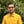Trusted answers to developer questions
Trusted Answers to Developer Questions

Related Tags

d
floor
math
communitycreator

# What is the floor() function in D?Harris Amjad

Grokking Modern System Design Interview for Engineers & Managers

Ace your System Design Interview and take your career to the next level. Learn to handle the design of applications like Netflix, Quora, Facebook, Uber, and many more in a 45-min interview. Learn the RESHADED framework for architecting web-scale applications by determining requirements, constraints, and assumptions before diving into a step-by-step design process.

### Overview

The floor() function in D returns the next largest integer that is less than or equal to a specified number.

Figure 1 shows the mathematical representation of the floor() function.

Figure 1: Mathematical representation of the "floor()" function

Note: We need to import std.math in our code in order to use the floor() function. We can import it like this:
import std.math

### Syntax

floor(number)
// number should be float, double or real.


### Parameter

This function requires a number as a parameter.

### Return value

The floor() function returns the next largest integer that is less than or equal to the number set as a parameter.

Note: If -0.0, real.infinity, -real.infinity, real.nan, or -real.nan is sent as a parameter, then the function returns the parameter as it is.

### Code example

The code written below demonstrates the use of the floor() function in D:

import core.stdc.stdio;import std.stdio;//header required for functionimport std.math;int main(){    //integer    writeln ("The value of floor(4.0) : ",floor(4.0));    //positive double value    writeln ("The value of floor(2.56) : ",floor(2.56));    //negative double value    writeln ("The value of floor(-2.56) : ",floor(-2.56));    //exceptional outputs    writeln ("The value of floor(-0.0) : ",floor(-0.0));    writeln ("The value of floor(real.infinity) : ",floor(real.infinity));    writeln ("The value of floor(-real.infinity) : ",floor(-real.infinity));    writeln ("The value of floor(real.nan) : ",floor(real.nan));    writeln ("The value of floor(-real.nan) : ",floor(-real.nan));    return 0;}

### Code explanation

• Line 4: We add the header std.math, which is required for the floor() function.

• Line 9: We calculate the floor of the integer value, using the floor() function.

• Line 12: We calculate the floor of the positive double value, using the floor() function.

• Line 15: We calculate the floor of the negative double value, using the floor() function.

• Lines 18–25: We calculate the floor of the exceptional numbers, using the floor() function.

RELATED TAGS

d
floor
math
communitycreator

CONTRIBUTORHarris Amjad

Grokking Modern System Design Interview for Engineers & Managers

Ace your System Design Interview and take your career to the next level. Learn to handle the design of applications like Netflix, Quora, Facebook, Uber, and many more in a 45-min interview. Learn the RESHADED framework for architecting web-scale applications by determining requirements, constraints, and assumptions before diving into a step-by-step design process.

Keep Exploring

Learn in-demand tech skills in half the time